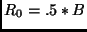Next: TCP-Friendliness Up: Evaluation Previous: Behavior of Uncoordinated TCP

## Behavior of C-TCP Flows

We postulate here that use of the Coordination Protocol (CP) should be distinctive in at lease two ways. First, since all flows make use of the same bandwidth availability calculation, round trip time, and loss rate information, bandwidth usage patterns among CP flows should be much smoother. That is, there should be far fewer jagged edges and less criss-crossing of individual flow bandwidths as flows need not search the bandwidth space in isolation for a maximal send rate.

Second, the use of bandwidth by a set of CP flows should reflect the priorities and configuration of the application-including intervals of congestion when network resources become limited.

To test these hypotheses, we implemented three simple bandwidth sharing schemes which reflect different objectives an application may wish to achieve on an aggregate level. We note here that more schemes are possible, and the mixing of schemes in complex, application-specific ways is an open area of research.Figure 8 shows a simple equal bandwidth sharing scheme in which C-TCP flows divide available bandwidth () equally among themselves. (whereis the send rate for sending endpoint, andis the number of sending endpoints.) The aggregate plot line shows the total bandwidth used by the multi-flow application at a given time instant. While not plotted on the same graph, this line closely corresponds to bandwidth availability values calculated by APs and communicated to cluster endpoints.

Figure 8 confirms our hypothesis that usage patterns among CP flows should be far smoother, and avoid the jagged criss-crossing effect seen in Figure 7. This is both because flows are not constantly trying to ramp up in search of a maximal sending rate, and because of the use of weighted averages in the bandwidth availability calculation itself. The latter has the effect of dampening jumps in value from one instant to the next.Figure 9 shows a proportional bandwidth sharing scheme among C-TCP flows. In this particular scheme, flow 0 is configured to take .5 of the bandwidth (), while flows 1 and 2 evenly divide the remaining portion for a value of .25 each ().

Figure 9 confirms our second hypothesis above by showing sustained proportional sharing throughout the entire time interval. This includes the congestion intervals (times 5.0-8.0 and 14.0-20.0) and post-congestion intervals (times 8.0-10.0, 20.0-25.0) when TCP connections might still contend for bandwidth.

In Figure 10, we see a constant bandwidth flow in conjunction with two flows equally sharing the remaining bandwidth. The former is configured to send at a constant rate of 3.5 Mb/s or, if it is not available, at the bandwidth availability value for that given instant. (). Flows 1 and 2 split the remaining bandwidth or, if none is available, send at a minimum rate of 1Kb/s. ()

We observe that flows 1 and 2 back off their sending rate almost entirely whenever flow 0 does not receive its full share of bandwidth. We also note that while flow 0 is configured to send at a constant rate, it never exceeds available bandwidth limitations during time of congestion.

We emphasize once again the impossibility of achieving results like Figure 9 and Figure 10 in an application without the transport-level coordination provided by CP.Next: TCP-Friendliness Up: Evaluation Previous: Behavior of Uncoordinated TCP
David Ott 2002-04-16